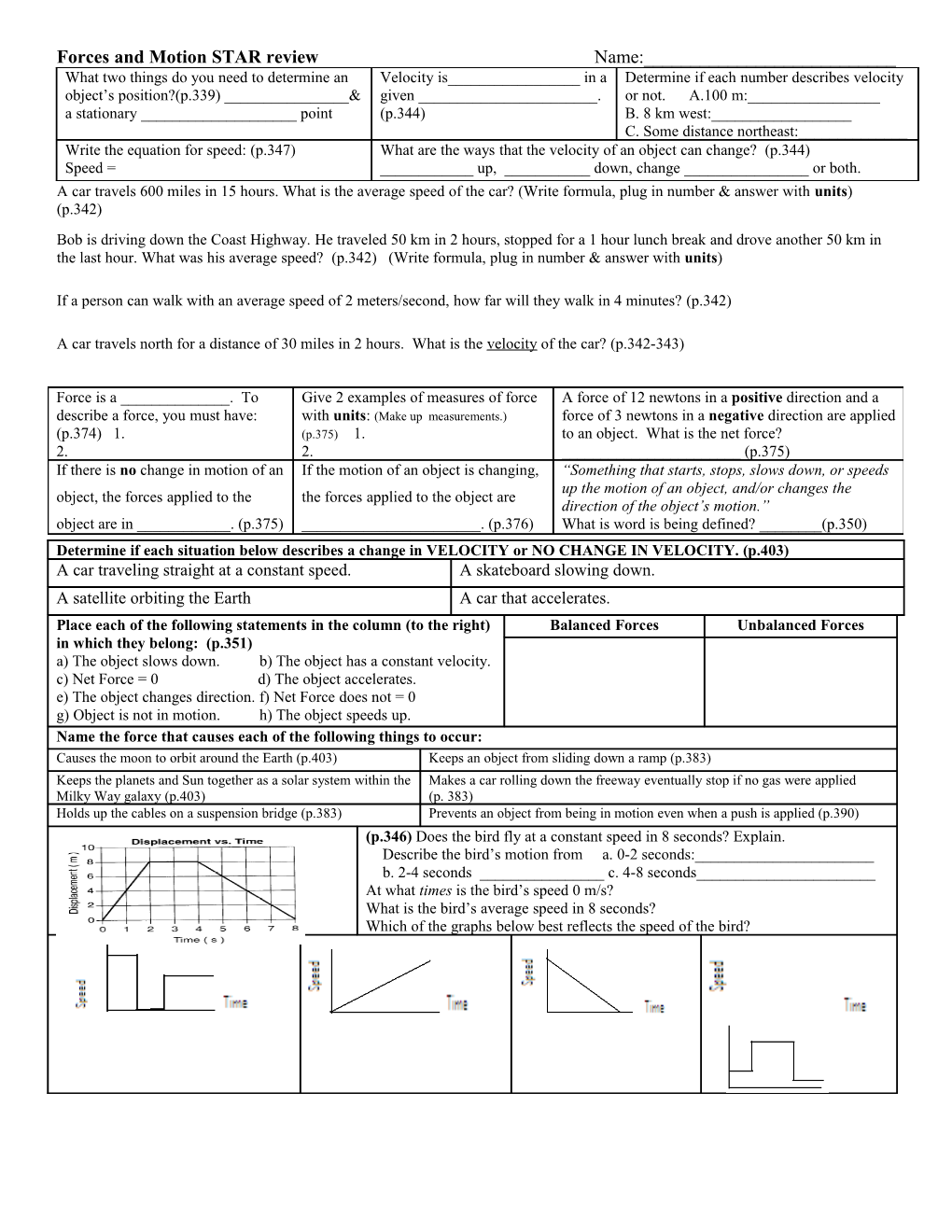# Motion Study GuideForces and Motion STAR review Name:______

What two things do you need to determine an object’s position?(p.339) ______& a stationary ______point / Velocity is______in a given ______.
(p.344) / Determine if each number describes velocity or not. A.100 m:______
B. 8 km west:______
C. Some distance northeast:______
Write the equation for speed: (p.347)
Speed = / What are the ways that the velocity of an object can change? (p.344)
______up, ______down, change ______or both.

A car travels 600 miles in 15 hours. What is the average speed of the car? (Write formula, plug in number answer withunits) (p.342)

Bob is driving down the Coast Highway. He traveled 50 km in 2 hours, stopped for a 1 hour lunch break and drove another 50 km in the last hour. What was his average speed? (p.342) (Write formula, plug in number answer withunits)

If a person can walk with an average speed of 2 meters/second, how far will they walk in 4 minutes?(p.342)

A car travels north for a distance of 30 miles in 2 hours. What is the velocity of the car? (p.342-343)

Force is a ______. To describe a force, you must have: (p.374) 1.
2. / Give 2 examples of measures of force with units:(Make up measurements.) (p.375) 1.
2. / A force of 12 newtons in a positive direction and a force of 3 newtons in a negative direction are applied to an object. What is the net force?______(p.375)
If there is no change in motion of an object, the forces applied to the object are in ______. (p.375) / If the motion of an object is changing, the forces applied to the object are ______. (p.376) / “Something that starts, stops, slows down, or speeds up the motion of an object, and/or changes the direction of the object’s motion.”
What is word is being defined? ______(p.350)
What would happen to the speed of an object if you: (p.376-377)
Increased the object’s mass: ______/ -Increase force in the same direction of motion:______
-Increase force in opposite direction of motion:______/ -Increased the force on the object in the same direction:______
-Increased the force on the object in the opposite direction:______
Decreased the object’s mass:______
Determine if each situation below describes a change in VELOCITY or NO CHANGE IN VELOCITY. (p.403)
A car traveling straight at a constant speed. / A skateboard slowing down.
A satellite orbiting the Earth / A car that accelerates.
Place each of the following statements in the column (to the right) in which they belong: (p.351)
a) The object slows down. b) The object has a constant velocity.
c) Net Force = 0 d) The object accelerates.
e) The object changes direction. f) Net Force does not = 0
g) Object is not in motion. h) The object speeds up. / Balanced Forces / Unbalanced Forces
Name the force that causes each of the following things to occur:
Causes the moon to orbit around the Earth (p.403) / Keeps an object from sliding down a ramp (p.383)
Keeps the planets and Sun together as a solar system within the Milky Way galaxy (p.403) / Makes a car rolling down the freeway eventually stop if no gas were applied
(p. 383)
Holds up the cables on a suspension bridge (p.383) / Prevents an object from being in motion even when a push is applied (p.390)
/ (p.346)Does the bird fly at a constant speed in 8 seconds? Explain.
Describe the bird’s motion from a. 0-2 seconds:______
b. 2-4 seconds ______c. 4-8 seconds______
At what times is the bird’s speed 0 m/s?
What is the bird’s average speed in 8 seconds?
Which of the graphs below best reflects the speed of the bird?